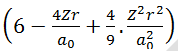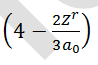# In a hydrogen like sample electron is in 2nd excited state, the Binding energy of 4th state of this sample is 13.6 eV, then(A)    A 25 eV photon can set free the electron from the second excited state of this sample.(B)    3 different types of photon will be observed if electrons make transition up to ground state from the second excited state(C)    If 23 eV photon is used then K.E. of the ejected electron is 1 eV.(D)    2nd line of Balmer series of this sample has same energy value as 1st excitation energy of H-atom.Aditi Chauhan
9 years ago

(A, B, D)

3s orbital has two radial node at the values of radius given by solutions of= 0

3pz orbital has on radial nodal surface at= 0

for    3pz,          at r = 0             y = 0 while for

3s             at r = 0             y = maximum so, 3s has greater                                   penetrating power than 3pz orbital